# Analysis of Proportions

Study size calculations for analyses involving proportions are functions of the overall study size, the proportion of participants in each of the comparison groups and the difference between the expected proportion of events in the different groups (9). A study size calculation for a two-group comparison of proportions has the following formula: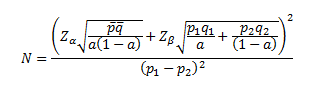In the above equation, p1 is the probability that someone with the characteristic will have a particular event, p2 is the probability that someone without the characteristic will have the event,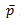is the weighted average (weighted by a) of p1 and p2, and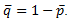.

p~ Studies to compare two groups that include most participants (for example N=1600 and “a” near 0.5) can have low values of "a" (e.g., 0.1) and low probabilities of outcomes (e.g., 0.1) and still have adequate power to detect relative risks of 2 (93%). However, if a subgroup analysis of 300 participants is performed (such as might be the case if two groups were being compared that only differed with respect to the timing of disease progression), both "a" and the probability of and outcome would have to be higher (e.g., "a" = 0.3, probability of an outcome = 0.2, power = 0.94 to detect a relative risk of 2.0).

Below we have presented two figures showing the power to detect specified alternatives of p1 and p2 assuming that equal numbers of specimens are used in each group, and the proportion of specimens with an attribute (the control event rate) is low (0.1) or moderate (0.3). In both of these figures, we have assumed that the proportion of case specimens with the attribute (the case event rate) will be higher in the alternative. As can be seen from these figures, small sample sizes will be sufficient to detect large differences between event rates. If smaller differences between the two event rates are postulated in the alternative hypothesis, larger study sizes will be necessary.

Comparison of Two Proportions

Control Event Rate Low (0.1)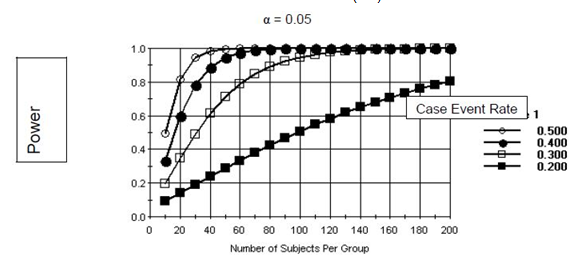Comparison of Two Proportions

Control Event Rate Moderate (0.3)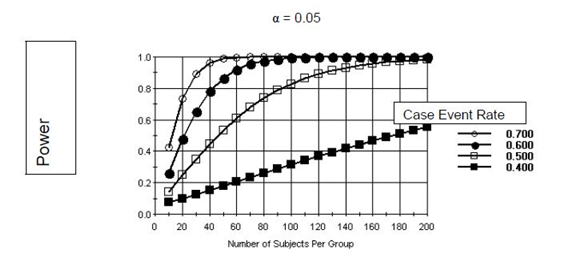## Horizontal Tabs

This page is associated with a larger collection of pages. You can view them as a whole here.